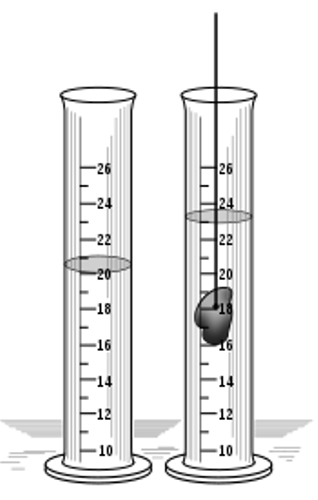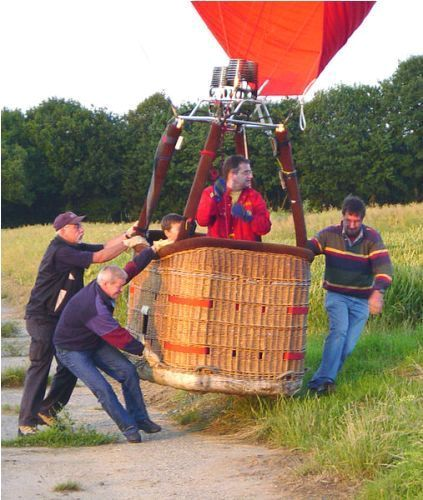# 10 Fun Facts about Mass and Weight

Post On: July 27, 2017
By: Agustina

Fun Facts about Mass and Weight talk about two important concepts in science. Mass and weight are different. The common people often use the term and weight interchangeably. However, actually both have different quantities and concepts. The force of an object by the gravity is defined as weight. On the other hand, mass is defined as the amount of matter in an object. The weight of an object at 9.81 Newton in the earth’s surface has the mass of 1 kilogram.  Let us get other interesting facts about mass and weight by reading the following post:

## Fun Facts about Mass and Weight 1: measurement

A unit of mass is measured in kilogram. A unit of force in Newton used to measure the weight.

### Fun Facts about Mass and Weight 2: gravity

Gravity affects the weight of an object. When the gravity is weaker, the weight of an object will be less. For instance, the weight of a person in Mars is lesser than on earth. However, the mass of a person is always the same.## Fun Facts about Mass and Weight 3: how to measure the weight

Is it easy to measure the weight of a person? It is sometimes difficult for the people to do it. However, all objects located on the surface of earth have weight.

### Fun Facts about Mass and Weight 4: floating object

A small object floating on the water is buoying which makes you think that it does not have any weight. Actually, it is not true.  This object transfers its weight into the bottom part of the container.

Related Article: 10 Facts about Liquids

## Fun Facts about Mass and Weight 5: a balloon

A balloon on the air seems weightless even though it has mass. The weight of this object is difficult to measure because the weight is transferred into Earth’s surface.Mass and Weight Image

### Fun Facts about Mass and Weight 6: the factors

The gravitational field is the primary factor, which affects the weight of an object.

## Fun Facts about Mass and Weight 7: a constant mass

The mass of an object is always constant as long as the object does not receive additional matter or energy.

### Fun Facts about Mass and Weight 8: the importance of mass and weight

Mass and weight are important in commerce. The use of mass and weight have been defined by NIST.Mass and Weight

## Fun Facts about Mass and Weight 9: the handbook

The handbook 130 of NIST defines the regulation and laws of mass and weight for engine fuel quality and legal meteorology.

Check Also: 10 facts about Luis Walter Alvarez

### Fun Facts about Mass and Weight 10: the content of handbook 130

The handbook 130 contains the explanation about the definition, regulation, use of terms or mass and weight.

Do you like learning facts about mass and weight?